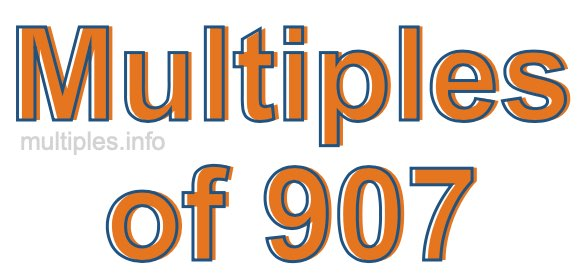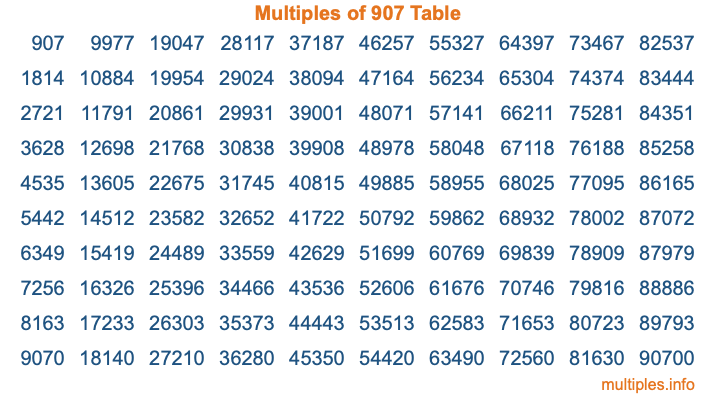Multiples of 907Welcome to the Multiples of 907 page. Here we will first teach you everything you will ever need to know about the multiples of 907, and then give you a study guide summary of everything we taught you to make sure you remember it all. Use this page to look up facts and learn information about the multiples of 907. This page will make you a multiples of nine hundred seven expert!

Definition of Multiples of 907
Multiples of 907 are all the numbers that when divided by 907 equal an integer. Each of the multiples of 907 are called a multiple. A multiple of 907 is created by multiplying 907 by an integer.

Therefore, to create a list of multiples of 907, you start with 1 multiplied by 907, then 2 multiplied by 907, then 3 multiplied by 907, and so on for as long as you want. Thus, the list of the first five multiples of 907 is 907, 1814, 2721, 3628, and 4535. To see a larger list of multiples of 907, see the printable image of Multiples of 907 further down on this page. We also have a category where you can choose any nth multiple of 907.

Multiples of 907 Checker
The Multiples of 907 Checker below checks to see if any number of your choice is a multiple of 907. In other words, it checks to see if there is any number (integer) that when multiplied by 907 will equal your number. To do that, we divide your number by 907. If the the quotient is an integer, then your number is a multiple of 907.

Is  a multiple of 907?

Least Common Multiple of 907 and ...
A Least Common Multiple (LCM) is the lowest multiple that two or more numbers have in common. This is also called the smallest common multiple or lowest common multiple and is useful to know when you are adding our subtracting fractions. Enter one or more numbers below (907 is already entered) to find the LCM.

Check out our LCM Calculator if you need more details about the Least Common Multiple or if you need the LCM for different numbers for adding and subtraction fractions.

nth Multiple of 907
As we stated above, 907 is the first multiple of 907, 1814 is the second multiple of 907, 2721 is the third multiple of 907, and so on. Enter a number below to find the nth multiple of 907.

th multiple of 907

Multiples of 907 vs Factors of 907
907 is a multiple of 907 and a factor of 907, but that is where the similarities end. All postive multiples of 907 are 907 or greater than 907. All positive factors of 907 are 907 or less than 907.

Below is the beginning list of multiples of 907 and the factors of 907 so you can compare:

Multiples of 907: 907, 1814, 2721, 3628, 4535, etc.

Factors of 907: 1, 907

As you can see, the multiples of 907 are all the numbers that you can divide by 907 to get a whole number. The factors of 907, on the other hand, are all the whole numbers that you can multiply by another whole number to get 907.

It's also interesting to note that if a number (x) is a factor of 907, then 907 will also be a multiple of that number (x).

Multiples of 907 vs Divisors of 907
The divisors of 907 are all the integers that 907 can be divided by evenly. Below is a list of the divisors of 907.

Divisors of 907: 1, 907

The interesting thing to note here is that if you take any multiple of 907 and divide it by a divisor of 907, you will see that the quotient is an integer.

Multiples of 907 Table
Below is an image of the first 100 multiples of 907 in a table. The table is in chronological order, column by column. The first column has the first ten multiples of 907, the second column has the next ten multiples of 907, and so on.The Multiples of 907 Table is also referred to as the 907 Times Table or Times Table of 907. You are welcome to print out our table for your studies.

Negative Multiples of 907
Although not often discussed or needed in math, it is worth mentioning that you can make a list of negative multiples of 907 by multiplying 907 by -1, then by -2, then by -3, and so on, to get the following list of negative multiples of 907:

-907, -1814, -2721, -3628, -4535, etc.

Multiples of 907 Summary
Below is a summary of important Multiples of 907 facts that we have discussed on this page. To retain the knowledge on this page, we recommend that you read through the summary and explain to yourself or a study partner why they hold true.

There are an infinite number of multiples of 907.

A multiple of 907 divided by 907 will equal a whole number.

907 divided by a factor of 907 equals a divisor of 907.

The nth multiple of 907 is n times 907.

The largest factor of 907 is equal to the first positive multiple of 907.

907 is a multiple of every factor of 907.

907 is a multiple of 907.

A multiple of 907 divided by a divisor of 907 equals an integer.

907 divided by a divisor of 907 equals a factor of 907.

Any integer times 907 will equal a multiple of 907.

Multiples of a Number
Here you can get the multiples of another number, all with the same attention to detail as we did for multiples of 907 on this page.

Multiples of
Multiples of 908
Did you find our page about multiples of nine hundred seven educational? Do you want more knowledge? Check out the multiples of the next number on our list!